Mathematics

# Euclidean Geometry : History, Axioms and Postulates

230 views

Table of Contents

 1 Introduction 2 History of Euclid - Father of Geometry 3 Euclid’s Elements 4 Euclid’s Axioms 5 Euclid’s Postulates 8 Frequently Asked Questions (FAQs)

15 December 2020

Read time: 3 minutes

## Introduction

The Study of Plane and Solid figures based on postulates and axioms defined by Euclid is called Euclidean Geometry.

Euclid is known as the father of Geometry because of the foundation of geometry laid by him.

Euclid realized that for a proper study of Geometry, a basic set of rules and theorems must be defined.

### Non-Euclidean Geometry

There is a branch of Geometry known as Non-Euclidean Geometry. Basically, it is everything that does not fall under Euclidean geometry.

However, it is commonly used to describe Hyperbolic Geometry.

### Euclidean Geometry: History, Axioms, and Postulates-PDF

Learn about Euclidean Geometry, the different Axioms, and Postulates with Exercise Questions. Here is a downloadable PDF to explore more.

 📥 Euclidean Geometry: History, Axioms, and Postulates-PDF Download

Also read,

## History of Euclid - Father of Geometry

Euclid was born around 325 B.C. He was a Greek Mathematician from Alexandria, also known as the Father of Geometry or the Founder of Geometry.

He did most of his work during the rule of Ptolemy (283 - 323 BC).

His work has had a profound influence on the mathematics studied then and the mathematics we learn today.

Most of his work revolved around Geometry, and his Elements, Axioms, and Postulates have helped shape geometry to be as it is today.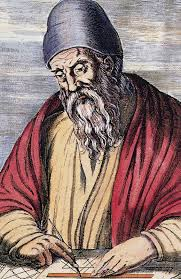## Euclid’s Elements

Euclid’s Elements are a set of 13 books written by Euclid.

They have been referred to as one of the most successful and influential textbooks.

It is one of the earliest mathematical books ever printed since the printing press’s invention in 1482.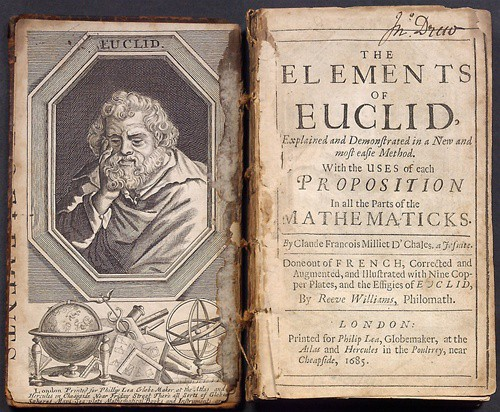Euclid’s Elements are estimated to be second in the volume of books published after the Bible.

They are an assembly of theorems, postulates, definitions, and proofs in mathematics.

They talk about Plane and Solid Euclidean Geometry, Incommensurable lines, and elementary number system.

## Euclid’s Axioms

Listed below are Euclid’s Axioms:

1. Things that are equal to the same thing are equal to one another.

2. If equals are added to equals, the wholes are equal.

3. If equals are subtracted from equals, the remainders are equal.

4. Things that coincide with one another are equal to one another.

5. The whole is greater than the part.

6. Things that are double of the same things are equal to one another.

7. Things that are halves of the same things are equal to one another.

## Euclid’s Postulates

### Euclid’s Postulate 1

“A straight line segment can be drawn joining any two points.”

For any two points, a line can be drawn such that it passes through both of them.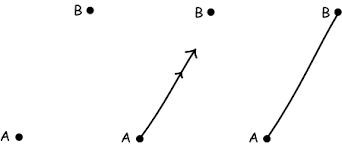Source: University of Pittsburgh

### Euclid’s Postulate 2

“To produce a finite straight line continuously in a straight line’”

A line segment can be extended in any direction to form a line.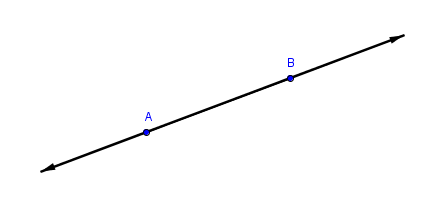### Euclid’s Postulate 3

“To describe a circle with any center and distance.”

A circle can be defined by taking any point as the center and having a specified radius.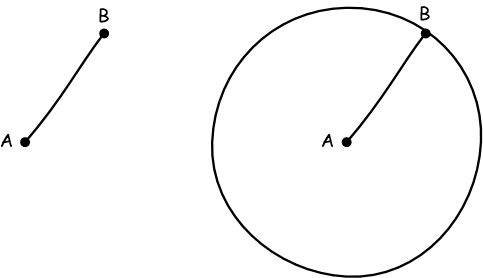Source: University of Pittsburgh

### Euclid’s Postulate 4

“That all right angles are equal to one another.”

Any angle that measures 90° is equal to another 90° angle, irrespective of the length of the lines forming the angles.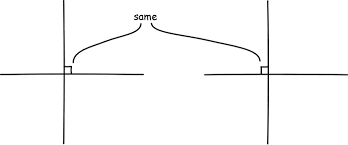Source: University of Pittsburgh

### Euclid’s Postulate 5

“If a straight line falling on two other straight lines makes the interior angles on the same side of it taken together less than two right angles, then the two straight lines, if produced indefinitely, meet on the side on which the sum of angles is less than two right angles.”

When there are two lines cut by a third line, if the interior angles on one side add up to less than 180°, then the two lines will meet when extended on that side.

In the image given below, $$\text{∠A}_1 + \text{∠B}_2 < 180°.$$

∴ Line m and n will meet when extended on the side of $$1$$ and $$2.$$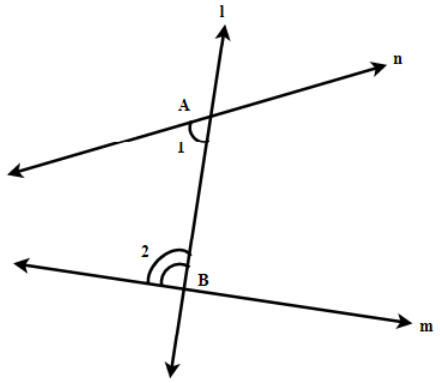## Conclusion

Euclid made some of the most important contributions in geometry.

To master geometry, one surely has to have good knowledge of Euclidean geometry.

You don't need to cram these postulates and axioms. You should try to get the meaning of each postulate as they have some of the very basic concepts around them.

If you want to know more about Euclid, click the link in the external references section.

Also read,

## About Cuemath

Cuemath, student-friendly mathematics and coding platform, conducts regular Online Live Classes for academics and skill-development, and their Mental Math App, on both iOS and Android, is a one-stop solution for kids to develop multiple skills.

Check out the fee structure for all grades and book a trial class today!

## Who is the Father of Geometry?

Euclid, an Ancient Greek Mathematician, is known as the Father of Geometry. He is also known as the Founder of Geometry.

## What are Euclid’s Postulates?

Let the following be postulated:

• To draw a straight line from any point to any point.

• To produce a finite straight line continuously in a straight line.

• To describe a circle with any center and distance.

• That all right angles are equal to one another.

• That if a straight line falling on two straight lines makes the interior angles on the same side less than two right angles, the straight lines, if produced indefinitely, will meet on that side on which the angles are less than two right angles.

## What is Non-Euclidean Geometry?

Literally, all geometry that is not a part of Euclidean Geometry is Non-Euclidean Geometry. However, it is commonly used to describe Hyperbolic Geometry.

## What are Euclid’s Axioms?

• Things that are equal to the same thing are equal to one another.

• If equals are added to equals, the wholes are equal.

• If equals are subtracted from equals, the remainders are equal.

• Things that coincide with one another are equal to one another.

• The whole is greater than the part.

• Things that are double of the same things are equal to one another.

• Things that are halves of the same things are equal to one another.

## External References

GIVE YOUR CHILD THE CUEMATH EDGE
Access Personalised Math learning through interactive worksheets, gamified concepts and grade-wise courses
Learn More About Cuemath